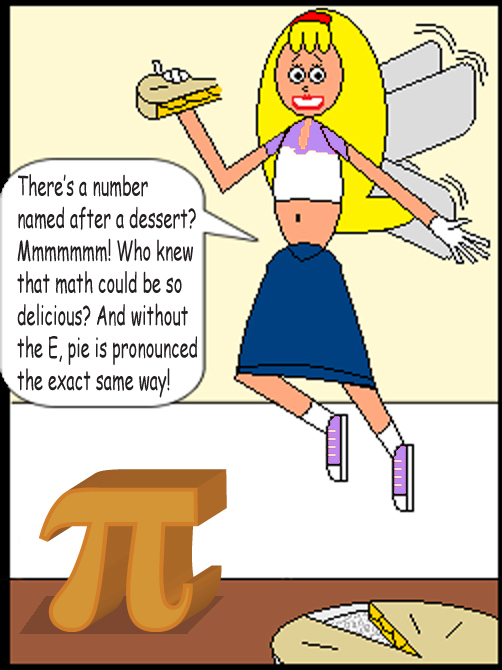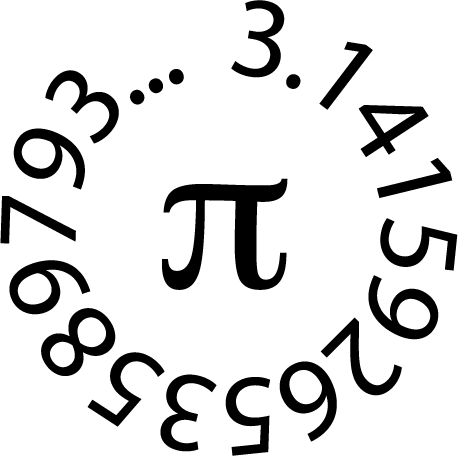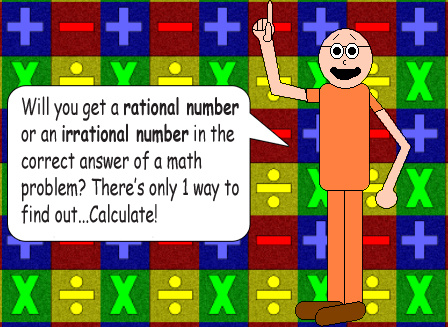# Pi(e): A Dessert & A Number!

### This famous irrational number is approximately equal to 3.14159. Although this number is irrational, some of its multiples are almost rational. The fractions below are approximations to the nearest billionths.## 37π ≈ 116 + 642/2687 or 312,334/2687

#### (To the left of the OR's above are the fractions as mixed numbers; I like to use the plus sign between the integer & the fraction!)

In the image below are the digits of Pi up to 15 digits after the decimal point:A very close rational number to Pi is 22/7 or 3 + 1/7. If you subtract Pi from that number, you get a very small number that's...

Therefore:

## π + 6/4,745 = 22/7 or 3 + 1/7

You can also print the difference as: (22 - 7π)/7.Note: Be warned, the TI-84 Plus sees no significance in adding non-integers smaller than a billionth(1/1,000,000,000 or 0.000000001) to integers! In fact, lots of calculators round up non-integers to the best of their display ability.